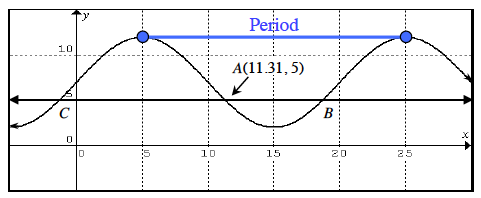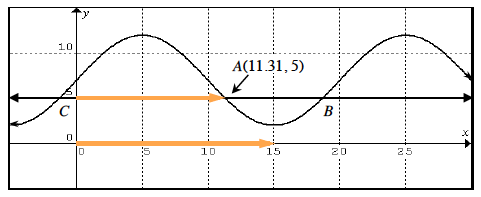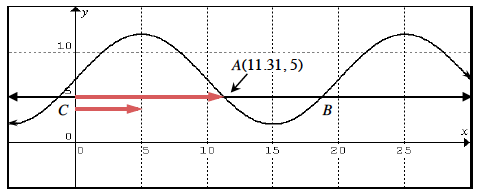### Home > PC > Chapter 6 > Lesson 6.2.2 > Problem6-84

6-84.
1. Below is a graph of a periodic function and the line y = 5. One of the intersection points is (11.31, 5). Homework Help ✎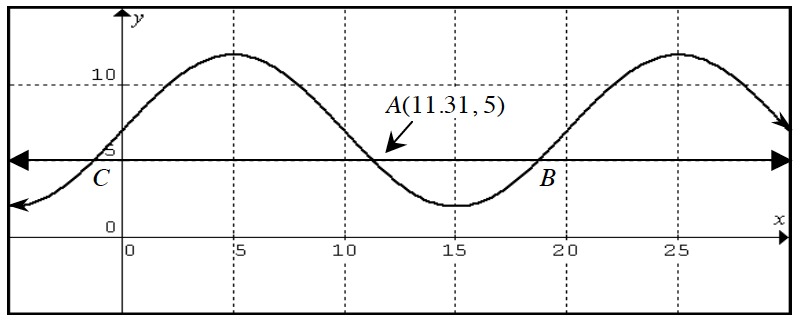1. What is the period of the function?

2. Use the symmetry of the graph to find the coordinate of the intersection labeled as point B.

3. What is the coordinate for the point labeled C?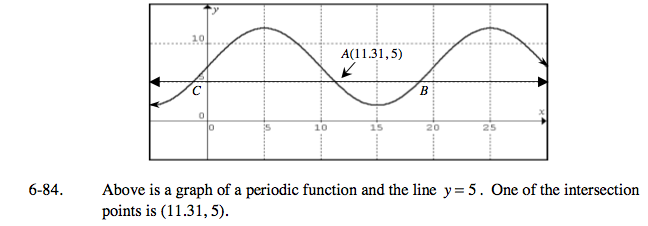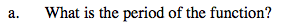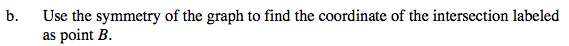Set up an equation involving B.
15 − 11.31 = B − 15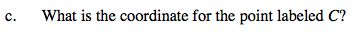Set up an equation involving C.
11.31 − 5 = 5 − C